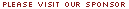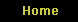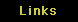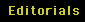### Gold & The Miners: Wave Counts Look Good

captainewave
website: www.captainewave.com

May 5, 2020

Gold: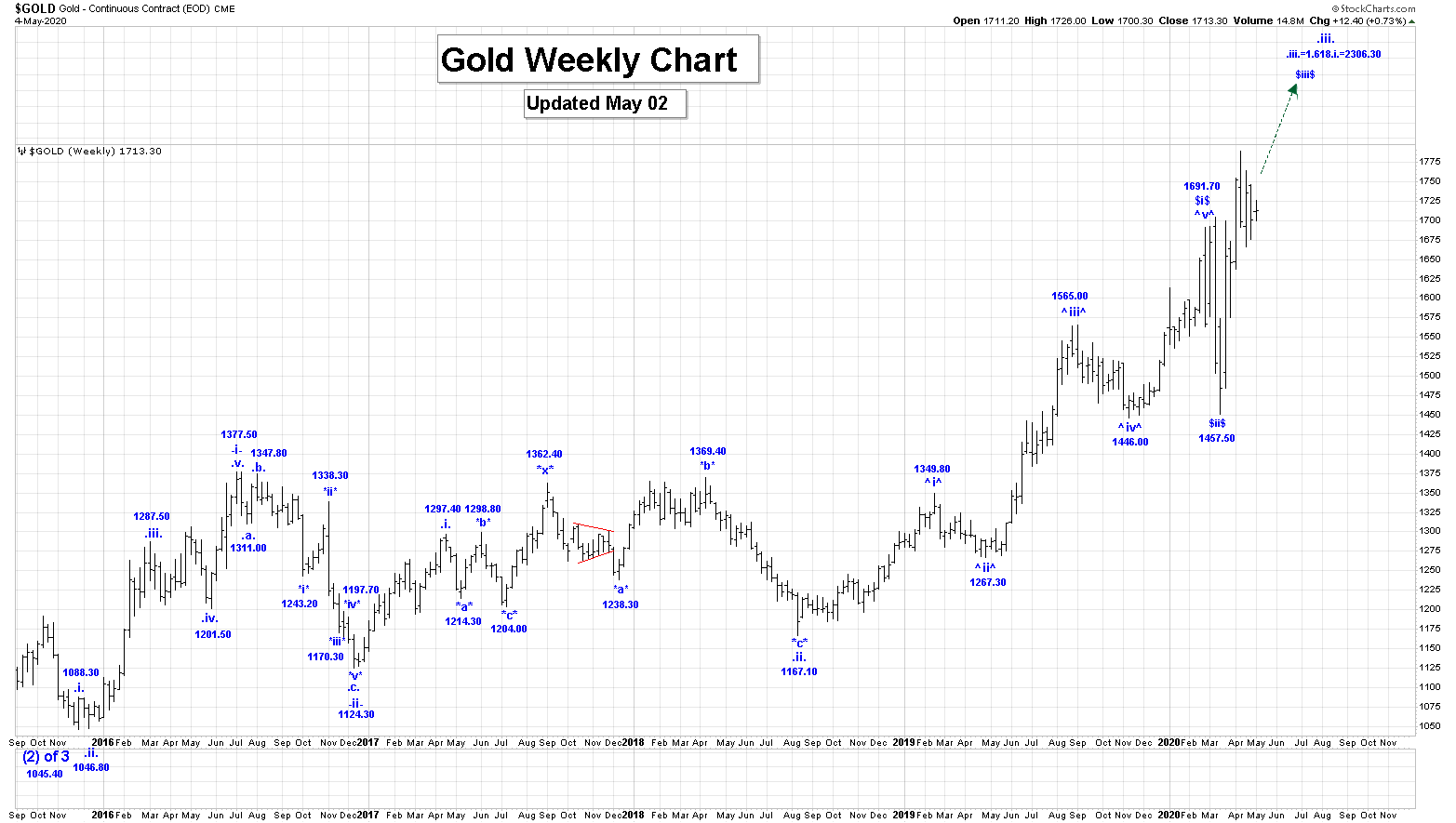Our current projection for the end of wave .iii. is:

.iii. = 1.618.i. = 2306.30.

Active Positions: Long with puts as stops!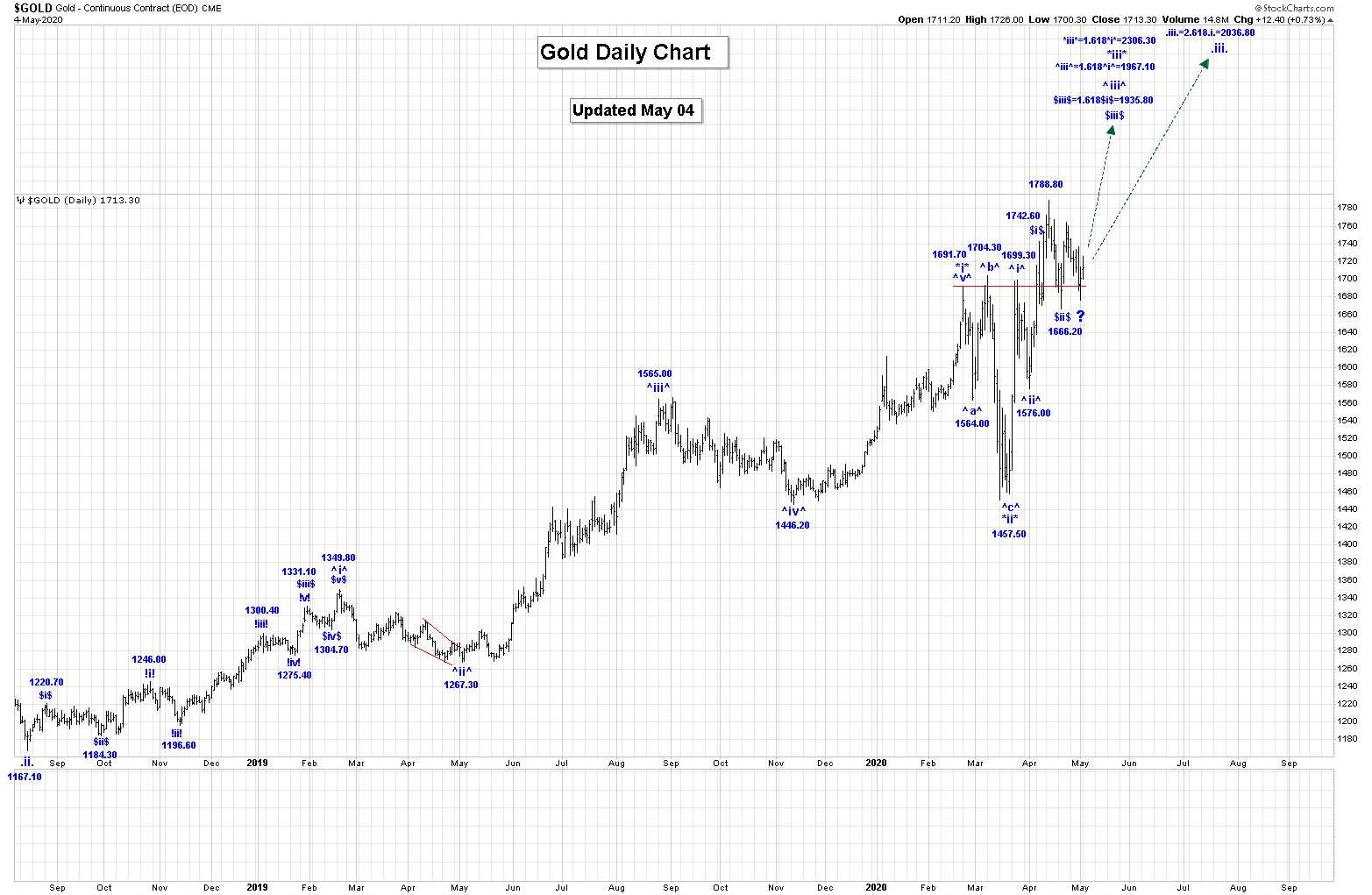Wave ^iii^ is now subdividing as shown on the daily chart, but we have now updated our internal count to suggest that all of wave \$i\$ ended at the 1742.60 high and all of wave \$ii\$ at the 1666.20 low.

We are now rallying in wave \$iii\$, which has the following initial projection for its completion:

\$iii\$ = 1.618\$i\$ = 1935.80.

Intraday, we appear to have corrective pattern in place from the 1763.60 high to the current low of 1676.40 level.

Although it has become very deep, which may be suggesting that all of wave \$ii\$ may not be complete at the 1666.20 low. Remember the current wave \$ii\$ low was about \$7 short of our 50% retracement level.

For now, we will stick with our current analysis and assume that all of wave \$ii\$ is complete at the 1666.20 low.

Once this correction ends, we expect gold to move higher again.

GDX & Gold Stocks: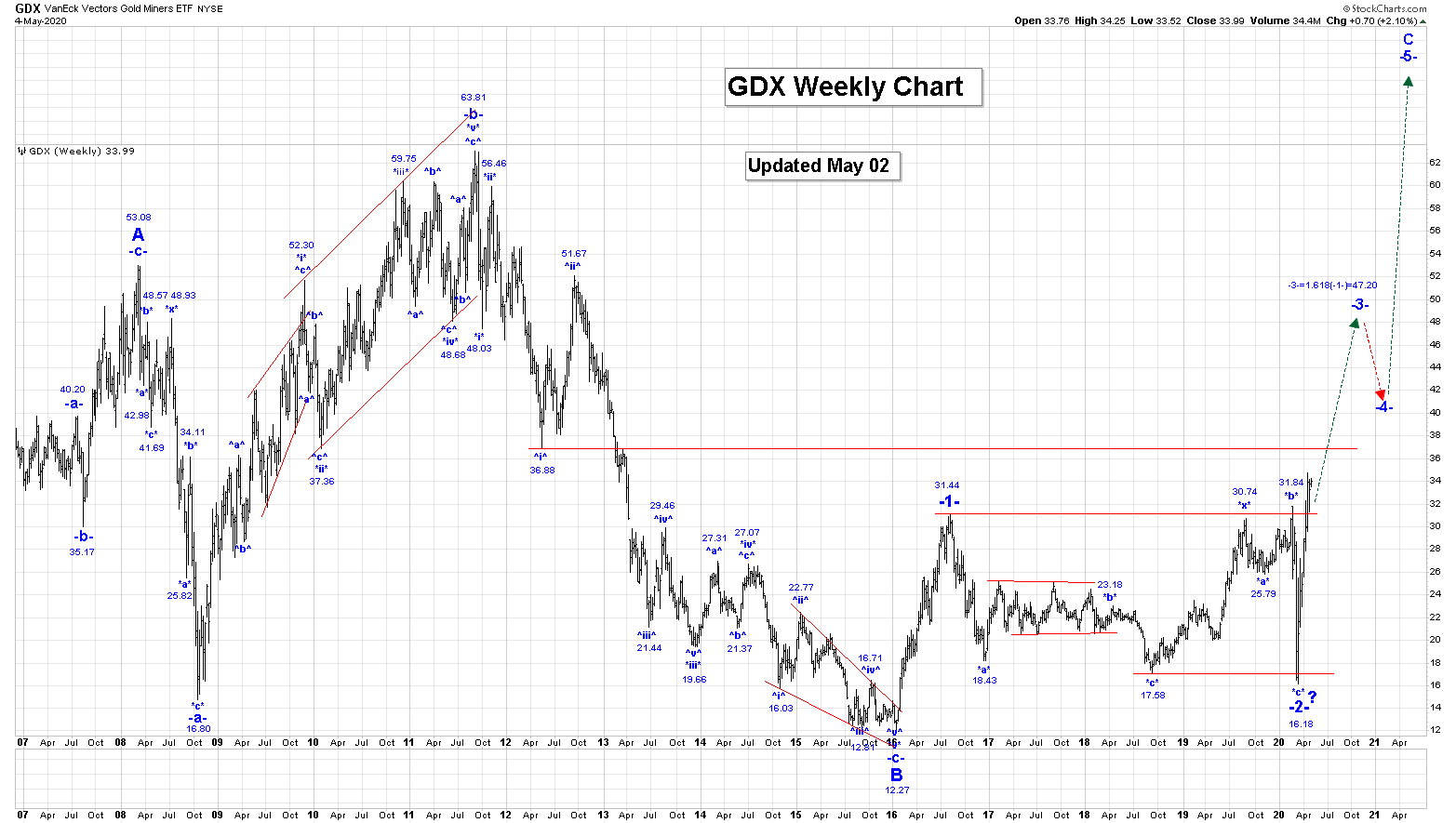Our first projection for the end of wave 3 is:

3 = 1.618 (1) = 47.20.

We suspect that wave 3 will extend higher than the above projection also, as gold is likely heading well above \$5000/oz.

We continue to rally sharply higher in wave -iii- for GDX, which has the following two projections for its completion: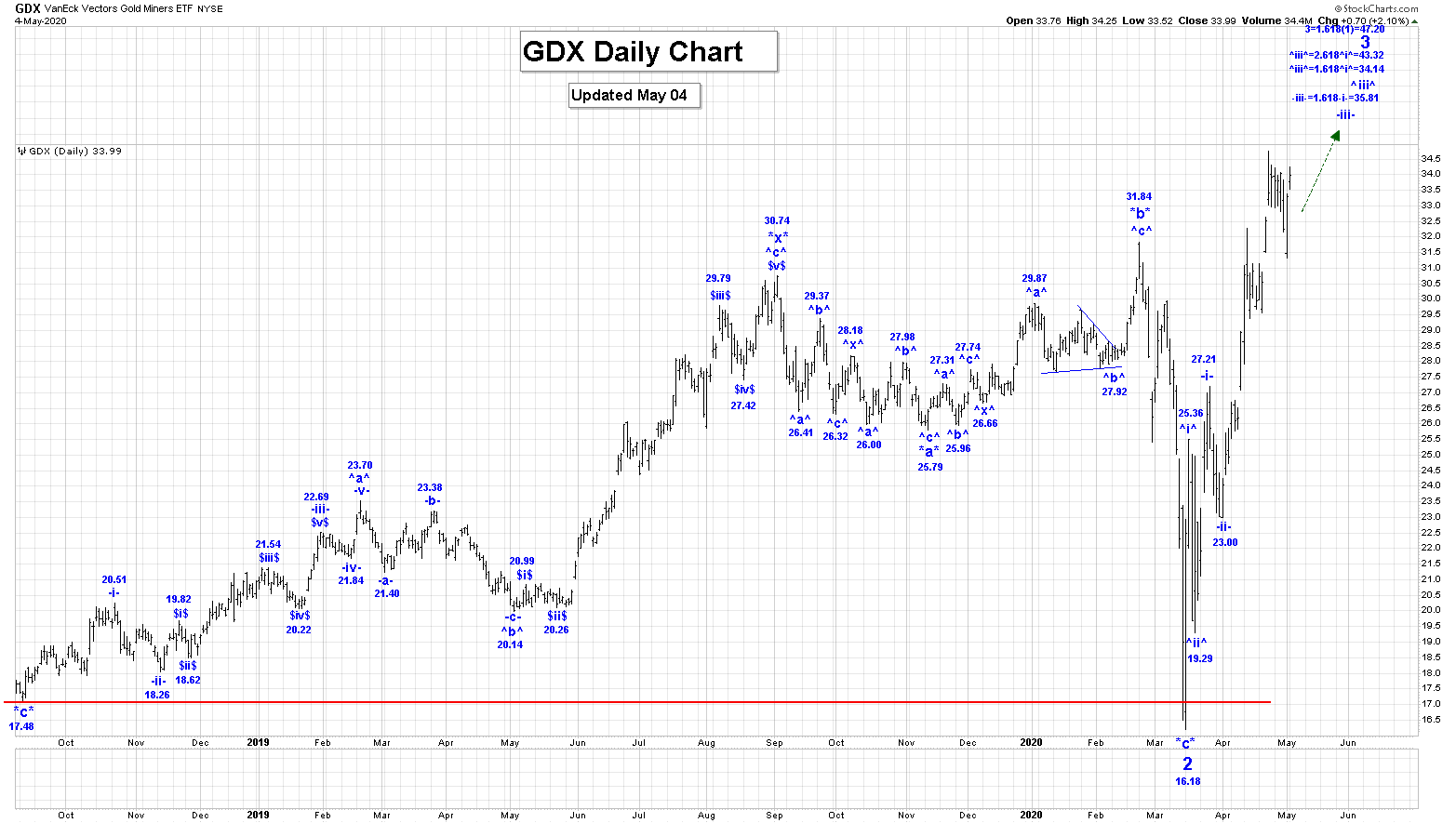-iii- = 1.618-i- = 35.81.

-iii- = 2.618-i- = 43.73.

We also continue to rally sharply higher in wave \$iii\$, which has the following initial projection for its completion:

\$iii\$ = 1.618\$i\$ = 37.45.

Within wave \$iii\$ we completed wave *i* at the 32.27 high and all of wave *ii* at the 29.55 low.

We should now be rallying sharply higher in wave *iii*, which has an initial projection for its completion of:

*iii* = 1.618*i* = 36.64.

We have updated our initial count for wave *iii* to suggest that wave (i) ended at 34.74 and likely all of wave (ii) at 31.31.

We should now be rallying higher in wave (iii) of *iii*.

Next resistance is at 34.74 and then 36.88. Our first projection for the end of wave (v) and *iii* is: 37.11!

Our first and second projections for the end of wave ^iii^ are:

^iii^ = 1.618^i^ = 34.14;

^iii^ = 2.618^i^ = 43.32.

We have updated our charts and counts for the following gold stocks and indices:

Kinross: We are now rallying in wave (iii), which has initial projection of 9.68, although we suspect that wave (iii) will extend in length as our second projection for its completion is:

(iii) = 2.618(i) = 14.19.

Within wave (iii) we are now rallying in wave -iii-, which has the following projections for its completion:

-iii- = 1.618-i- = 9.01;

-iii- = 2.618-i- = 12.90.

Barrick: We are now rallying in wave iii rally which has projected endpoints of:

Iii= 1.618i= 37.10;

Iii = 2.618i = 53.94

Within wave iii we are rallying sharply in wave (iii), which has projected endpoints of:

(iii) = 1.618(i) = 32.79;

(iii) = 2.618(i) = 45.24.

Newmont Goldcorp: We are now rallying in wave iii, which has projected endpoints of:

iii = 1.618i = 77.74;

iii = 2.618i = 107.85.

Within wave iii we are now rallying in wave (iii), which has the following projected endpoints:

(iii) = 1.618(i) = 70.97

(iii) = 2.618(i) = 94.44.

HUI: We are now rallying in wave 3 which has the following projected endpoints:

3 = 1.618(1) = 433.46;

3 = 2.618(1) = 620.32.

Within wave 3 we are rallying in wave iii, which has the following projected endpoints:

iii = 1.618i = 351.99;

iii = 2.618i = 481.46.

XAU: We are now rallying in wave (iii), which has the following projected endpoints:

(iii) = 1.618(i) = 163.16;

(iii) = 2.618(i) = 226.55.

Within wave (iii), we are rallying in wave -iii-, which has the following projected endpoints:

-iii- = 1.618-i- = 148.70;

-iii- = 2.618-i- = 201.84.

Trading Recommendation: Long Term hold of all gold stocks and indices.

Active Positions: We remain long the GDX, ABX, KGC, NEM, SSRM, and TSX:XGD with no stops!!

Free Offer For 321Gold Readers: Please send me an Email to admin@captainewave.com and I’ll send you free short and long term wave counts for oil, silver, & the CDNX junior miners index!

Thank-you!

###

May 5, 2020
captainewave & Crew!